# Power Spectral Density of Line Codes

The line codes discussed in the previous lecture generally not the best line codes to be used because all of these line codes have the form of pulses. As it is known, pulses have spectrums of the form of sinc functions. So, in theory, channels with infinite bandwidths are required to transmit any of the line codes discussed previously. To study the performance of a line code we need to consider the Power Spectral Density (PSD) of line codes. The reason for not being able to use the Fourier transform to find the spectrum of a line code is that the information signals that generate a line code is a stochastic (non–deterministic) signal, and the Fourier transform cannot be applied for non–deterministic signals. To study the spectrum of stochastic signals, we use the PSD, which shows the distribution of the signal power versus the frequency.

Consider the following Polar NRZ line code y(t). This signal can be decomposed into two signals, the information signal x(t) represented by a sequence of delta functions that have positive or negative areas depending on the corresponding bits (0’s or 1’s) that is convolved by a pulse signal p(t).

y (t ) = p(t )*x (t ).

The signal y(t) in the above relation is similar to the output that we obtain when we pass a signal x(t) through a filter with impulse response p(t) and frequency transform P(ω). So, the we can obtain y(t) using the following block diagram.

Using the same concept of the PSD, transmitting the signal y(t) through a channel with
impulse response c(t) and transfer function C(ω) as shown below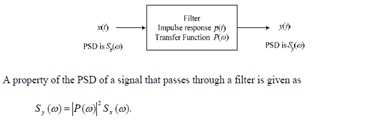Using the same concept of the PSD, transmitting the signal y(t) through a channel with impulse response c(t) and transfer function C(ω) as shown below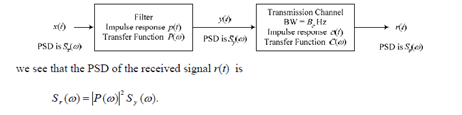Knowing that the PSD gives the spectrum of random signals (so the maximum frequency at which the PSD of a signal is non–zero can be considered as the bandwidth of that signal), we see that for a signal y(t) to be transmitted properly through a channel, the bandwidth of the channel Bc must at least be as much as the bandwidth of the transmitted signal y(t) (or the bandwidth of y(t) must be less than or equal to Bc).

This means that the line codes discussed in the previous lecture are in theory not suitable for most channels (and in practice they are not). The reason is that these line codes are built on pulses (similar to the rect function) so they have very high bandwidth. Unless the channel has a very high bandwidth to accommodate this wideband signal, part of the transmitted signal will be cutoff by the channel and the received signal will be different from the transmitted signal. When part of the spectrum of a transmitted signal is cutoff by the channel, a phenomenon known as Inter–Symbol Interference (ISI) occurs. When square pulses (which have high bandwidth) are transmitted, channels with limited bandwidth remove the high frequency components of the transmitted signal. This causes the pulse of every bit to extend beyond its boarders (instead of the pulse being confined to a bit period Tb, the pulse depending on how much was cut from the spectrum of the transmitted signal will extend its boundaries to several bit symbols. For some applications, this elongation of the pulse of a specific bit may effectively extend over 100 bits on each side. Clearly if every bit extends on each side over many bit periods, interference between the different bits will make it very difficult to detect the received bits. Therefore, the effect of ISI is that it will make it very difficult for the receiver to detect the transmitted bits. The extension of a pulse over many bit periods is shown in the next figure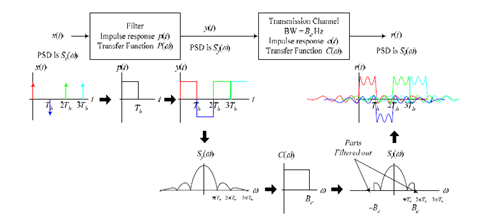It is clear from the above figure that the square pulses in y(t) got spread out in thereceived signal r(t). If the spreading is so severe, it may result in bit detection errors.

To reduce ISI in a signal, we need to change the PSD of the transmitted signal y(t). As seen above, Sy(ω) can be modified by either modifying Sx(ω) or modifying P(ω). Since we have no control over Sx(ω) because it is the PSD of the original information bits, we only can modify Sy(ω) by modifying P(ω), and hence modifying p(t), which is the pulse
used in the line code.

It is clear that if we use a pulse that looks like a sinc function, then its spectrum will be similar to a rect function. In fact, the sync function is in theory the best signal to be used in terms of the required bandwidth since its spectrum occupies the minimum band. The problem with sinc pulses is that they extend in time theoretically from t = –∞ to ∞ (in practice the sinc extends over a range around t = –100Tb to 100Tb depending on the requirements), so we would have to start generating a sinc pulse in theory at t = –∞ to transmit a bit at t = 0 and then continue generating this pulse until t = ∞. This is not
practical, so we will search for other pulses that are time–limited and do not cause ISI.

Nyquist Criterion for Zero–ISI

Nyquist proposed a condition for pulses p(t) to have zero–ISI when transmitted through a channel with sufficient bandwidth to allow the spectrum of all the transmitted signal to pass. Nyquist proposed that a zero–ISI pulse p(t) must satisfy the conditionA pulse that satisfies the above condition at multiples of the bit period Tb will result in zero–ISI if the whole spectrum of that signal is received. The reason for which these zero–ISI pulses (also called Nyquist–criterion pulses) cause no ISI is that each of these pulses at the sampling periods is either equal to 1 at the center of pulse and zero the points other
pulses are centered.

In fact, there are many pulses that satisfy these conditions. For example, any square pulse that occurs in the time period –Tb to Tb or any part of it (it must be zero at –Tb and Tb) will satisfy the above condition. Also, any triangular waveform (Δ function) with a width that is less than 2Tb will also satisfy the condition. A sinc function that has zeros at t =±Tb, ±2Tb, ±3Tb, … will also satisfy this condition. The problem with the sinc function is that it extends over a very long period pf time resulting in a lot of processing to generate it. The square pulse required a lot of bandwidth to be transmitted. The triangular pulse is restricted in time but has relatively large bandwidth.

There is a set of pulses known as raised–cosine pulses that satisfy the Nyquist criterion and require slightly larger bandwidth than what a sinc pulse (which requires the minimum bandwidth ever) requires.

The spectrum of these pulses is given by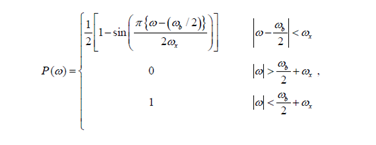where ωb is the frequency of bits in rad/s ( ωb = 2π/Tb), and ωx is called the excess bandwidth and it defines how much bandwidth would be required above the minimum bandwidth that is required when using a sinc pulse. The excess bandwidth ωx for this type of pulses is restricted between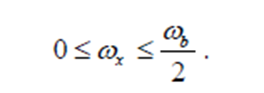Sketching the spectrum of these pulses we get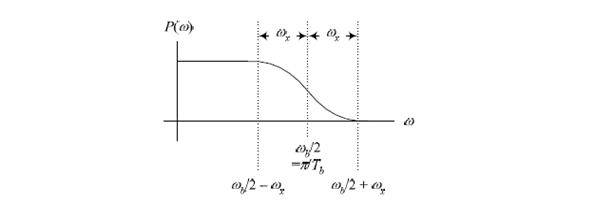We can easily verify that when ωx = 0, the above spectrum becomes a rect function, and therefore the pulse p(t) becomes the usual sinc function. For ωx = ωb/2, the spectrum is similar to a sinc function but decays (drops to zero) much faster than the sinc (it extends over 2 or 3 bit periods on each side). The expense for having a pulse that is short in time is that it requires a larger bandwidth than the sinc function (twice as much for ωx = ωb/2).Sketch of the pulses and their spectrum for the two extreme cases of ωx = ωb/2 and ωx = 0 are shown below.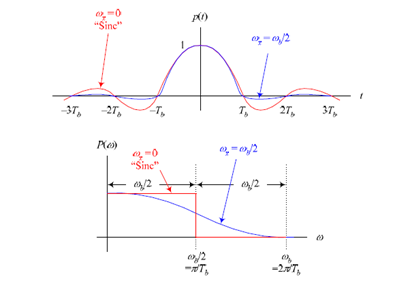We can define a factor r called the roll–off factor to be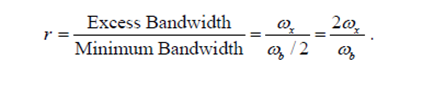The roll–off factor r specifies the ratio of extra bandwidth required for these pulses compared to the minimum bandwidth required by the sinc function.